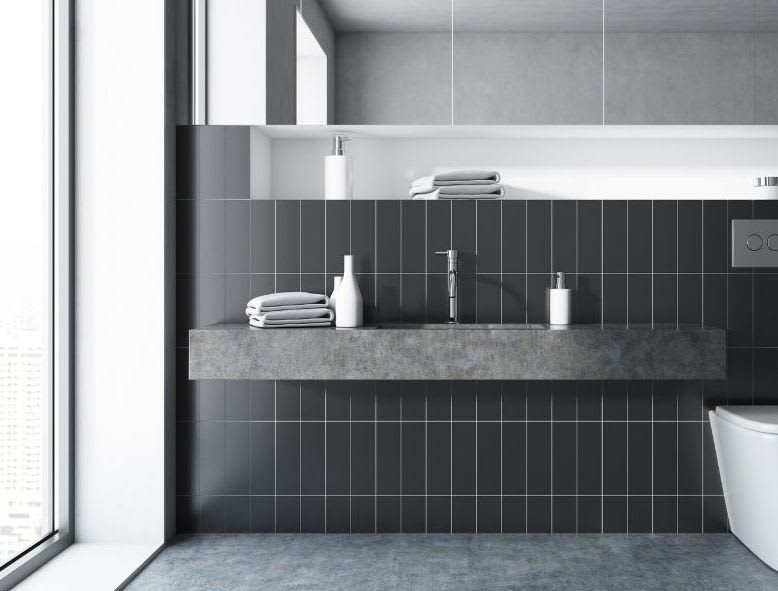Tiles are very popular-they are durable and easy to maintain. They come in different designs and patterns and are available in many sizes. However, accurately calculating the number of tiles you will need for your walls or floors can be complicated. A tile calculator makes this job a breeze.

This tool takes many factors into consideration while calculating the number of tiles required.  You need to furnish the following details:

• The width and length of the area that is to be covered with tiles
• Length and width of the tiles used
• The grout size or the grout lines (gaps between the tiles)

Once these figures are entered, the calculator lets you know how many tiles you will need and how many boxes (tiles usually come in boxes) you need to buy. The tool automatically takes into consideration factors like breakage of tiles and any other wastage.

The calculator can also be used to calculate the estimated cost of the tiles. You need to enter the price of a single tile. In case you have a box of tiles, mention the square footage the entire box covers. However, if you are using sheets, enter the size of the sheet.

Buy a few extra tiles-they will come in handy when broken tiles need to be replaced!Our tile calculator and tile estimator calculate tiles needed for any room or wall that you may have. Measurement for tiles can be tricky but if you follow our measuring for tiles guide you really can not go wrong. This tile calculator suits Australia and the tile calculator suit UK as well.

It a simple tile calculator to use and built by a Quantity Surveyor who does this type of work day in day out. You can rest assured we will calculate the amount of tile needed accurately and give you the tiles per square meter coverage.

Watch this tile calculator video below where I show you exactly how to use the calculator and explain what the calculation results mean.## Where can our tile calculator be used on?

• floor tile calculator
• bathroom tile calculator
• kitchen tile calculator
• shower tile calculator
• tile calculator floors
• tile calculator walls
• kitchen tile calculator
• garage floor tile calculator
• ceramic tile calculator

## What does our tile calculator do?

• calculate tiles required
• calculate the number of tiles per square meter
• calculate the number of tiles needed

## What our tile calculator estimate?

• ceramic tile cost estimates
• kitchen tile cost estimates
• floor tile cost estimates
• tile shower cost estimate

Watch this video below where I show you exactly how to use the calculator and explain what the calculation results mean.

Measuring wall tiles is as simple as multiplying the length by the height to give you the square meters. Then you remove your deductions for doors, windows, and openings. This then gives you the total square meters for your area. Add some wastage on top and you have the area you will need to purchase.

In the example video, I show you how to do that quickly using the excel spreadsheet. Once you have worked out the quantities you need for the project, you can work out the cost of the job by finding out what the materials price is. Use your local tiles store or DIY warehouse to find out the cost of the tiling materials you need.

The tile estimator will tell you at the bottom, the total cost of the tiling and the total cost per square meter so you can compare different tiling options you might be thinking about. Generally, wall tiles and floor tiles are different so that is why we have two separate calculators for you to use, again so you can compare.

Something you need to also consider is that during a renovation when removing tiles from a wall you may damage the wall itself. This would mean you need to allow for the cost of removing the tiles, disposing of the tiles and possibly re-patching the walls in places where necessary. Other costs could be for waterproofing under the tiles if they are in a wet area.